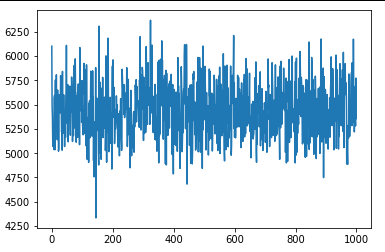# Trouble understanding losses from SVI in LDA extension

Hi,

Using pyro 0.5.1 , I’m trying to implement this LDA extension found in this paper
(https://beenkim.github.io/papers/KimRudinShahNIPS2014.pdf)

The model is described by this process:

I tried to implement a slightly simpler version of it - based on the lda example in the docs.
(only using \omega_j instead of \omega_{sj})

However, after running the SVI optimization step I’m not seeing any change in the loss (varying the learning rate didn’t seem to help). Any hints on where this may be going wrong would be greatly appreciated!import torch
import pyro
import pyro.distributions as dist
from pyro.infer import SVI, Trace_ELBO,TraceEnum_ELBO,config_enumerate
import torch.distributions.constraints as constraints
import numpy as np
import pyro.poutine as poutine
from pyro.infer import Predictive
import pandas as pd

from pyro.infer import MCMC, NUTS
import matplotlib.pyplot as plt

num_clusters = 5
num_features = 5
num_clusterings = 2
max_fval = 2
q = 0.4
lam = 2
c = 20

#Data read here is 56 data points x 147 feature, each is either 0 or 1

(num_points, num_features) = data.shape
num_vals_per =  * num_features # looking at binary features only anwaysy
max_fval = 2 # Only 2 possivle values for every feature

pyro.enable_validation(True)

def g(psj, wsj, lam, c, val):
return lam * (1 +  c * ((wsj == 1) & (psj == val)))

def model_bcm(data):
with pyro.plate("num_clusters", num_clusters):
proto_index = pyro.sample("prototypes", dist.Categorical(torch.ones(num_points) / num_points))

# for true bcm indent this line in
with pyro.plate("num_features", num_features):
subspace_feature = pyro.sample("subspace_feature", dist.Bernoulli(q))

with pyro.plate("num_features2", num_features) as fval:
with pyro.plate("num_cluster2s", num_clusters) as nclus:
prototypes_val = data[proto_index[nclus]]
g_out = torch.zeros(num_clusters,num_features,max_fval)
for v in range(max_fval):
#TODO Subspace feature be more explict
g_out[:,:,v] = lam * c * ((prototypes_val==v) * subspace_feature) + lam * torch.ones(num_clusters, num_features)
phi_big = pyro.sample("phi", dist.Dirichlet(g_out))

with pyro.plate("num_data", num_points):
pis = pyro.sample("pis", dist.Dirichlet(torch.ones(num_clusters) / num_clusters))
with pyro.plate("words", num_features):
#Tried to config the enumerations (parallel) but couldn't get it to work
zij = pyro.sample("zij", dist.Categorical(pis))

#Lining up things proerly for index easy
indRep= torch.linspace(0, num_features - 1, num_features)
indRep = indRep.type(torch.int64)
indRep = indRep.repeat(num_points)
word_topics_flat = zij.reshape(-1)
#\Flattineing out
out_dist = phi_big[word_topics_flat, indRep].reshape(num_points, num_features, -1)
out_dist = torch.transpose(out_dist, 0, 1)
data = pyro.sample("xij", dist.Categorical(out_dist), obs = torch.transpose(data, 0, 1))

def guide_bcm(data):
prototypes_posterior = pyro.param(
"prototype_posterior",
lambda: torch.ones(num_points) / num_points,
constraint = constraints.simplex
)

with pyro.plate("num_clusters", num_clusters):
proto_index = pyro.sample("prototypes", dist.Categorical(prototypes_posterior))

# for true bcm indent this line in
with pyro.plate("num_features", num_features):
subspace_feature = pyro.sample("subspace_feature", dist.Bernoulli(q))

phi_big = torch.zeros(num_clusters, num_features, max_fval)
with pyro.plate("num_features2", num_features) as fval:
with pyro.plate("num_cluster2s", num_clusters) as nclus:
prototypes_val = data[proto_index[nclus]]
g_out = torch.zeros(num_clusters,num_features,max_fval)
for v in range(max_fval):
g_out[:,:,v] = lam * c * ((prototypes_val==v) * subspace_feature) + lam * torch.ones(num_clusters, num_features)
phi_big = pyro.sample("phi", dist.Dirichlet(g_out))

pis_distribution = pyro.param(
"pis_distribution",
lambda: torch.ones(num_points, num_clusters) / num_clusters,
constraint = constraints.simplex
)

with pyro.plate("num_data", num_points):
pis = pyro.sample("pis", dist.Dirichlet(pis_distribution))
with pyro.plate("words", num_features):
zij = pyro.sample("zij", dist.Categorical(pis))

plt.show(losses)